Mathematical Statistics - 2017 Past Year Paper

# Mathematical Statistics - 2017 Past Year Paper - IIT JAM

Test Description

## 60 Questions MCQ Test IIT JAM Past Year Papers and Model Test Paper (All Branches) - Mathematical Statistics - 2017 Past Year Paper

Mathematical Statistics - 2017 Past Year Paper for IIT JAM 2023 is part of IIT JAM Past Year Papers and Model Test Paper (All Branches) preparation. The Mathematical Statistics - 2017 Past Year Paper questions and answers have been prepared according to the IIT JAM exam syllabus.The Mathematical Statistics - 2017 Past Year Paper MCQs are made for IIT JAM 2023 Exam. Find important definitions, questions, notes, meanings, examples, exercises, MCQs and online tests for Mathematical Statistics - 2017 Past Year Paper below.
Solutions of Mathematical Statistics - 2017 Past Year Paper questions in English are available as part of our IIT JAM Past Year Papers and Model Test Paper (All Branches) for IIT JAM & Mathematical Statistics - 2017 Past Year Paper solutions in Hindi for IIT JAM Past Year Papers and Model Test Paper (All Branches) course. Download more important topics, notes, lectures and mock test series for IIT JAM Exam by signing up for free. Attempt Mathematical Statistics - 2017 Past Year Paper | 60 questions in 180 minutes | Mock test for IIT JAM preparation | Free important questions MCQ to study IIT JAM Past Year Papers and Model Test Paper (All Branches) for IIT JAM Exam | Download free PDF with solutions
 1 Crore+ students have signed up on EduRev. Have you?
Mathematical Statistics - 2017 Past Year Paper - Question 1

### The imaginary parts of the eigenvalues of the matrix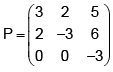are

Mathematical Statistics - 2017 Past Year Paper - Question 2

### Let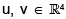be such that u = (1 2 3 5)T and v = (5 3 2 1)T. Then the equation uvT x = v has

Mathematical Statistics - 2017 Past Year Paper - Question 3

###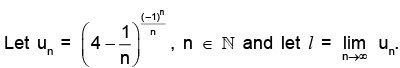Which of the following statements is TRUE ?

Mathematical Statistics - 2017 Past Year Paper - Question 4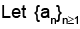be a sequence defined as follows :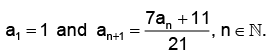Which of the following statements is TRUE ?

Mathematical Statistics - 2017 Past Year Paper - Question 5

Let X be a continuous random variable with the probability density function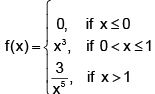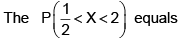Mathematical Statistics - 2017 Past Year Paper - Question 6

Let X be a random variable with the moment generating function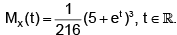Then P(X > 1) equals

Mathematical Statistics - 2017 Past Year Paper - Question 7

Let X be a discrete random variable with the probability mass function

p(x) = k(1 + |x|)2, x = –2, –1, 0, 1, 2,

where k is a real constant. Then P(X = 0) equals

Mathematical Statistics - 2017 Past Year Paper - Question 8

Let the random variable X have uniform distribution on the interval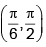. Then P(cos X > sin X) is

Mathematical Statistics - 2017 Past Year Paper - Question 9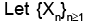be a sequence of i.i.d. random variables having common probability density function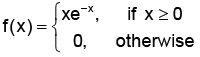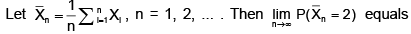Mathematical Statistics - 2017 Past Year Paper - Question 10

Let X1, X2, X3 be a random sample from a distribution with the probability density function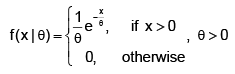Which of the following estimators of θ has the smallest variance for all θ > 0 ?

Mathematical Statistics - 2017 Past Year Paper - Question 11

Player P1 tosses 4 fair coins and player P2 tosses a fair die independently of P1. The probability that the number of heads observed is more than the number on the upper face of the die, equals

Mathematical Statistics - 2017 Past Year Paper - Question 12

Let X1 and X2 be i.i.d. continuous random variables with the probability density function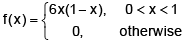Using Chebyshev’s inequality, the lower bound of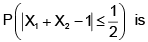Mathematical Statistics - 2017 Past Year Paper - Question 13

Let X1, X2, X3 be i.i.d. discrete random variables with the probability mass function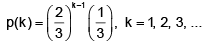Let Y = X1 + X2 + X3. Then P(Y > 5) equals

Mathematical Statistics - 2017 Past Year Paper - Question 14

Let X and Y be continuous random variables with the joint probability density function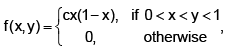where c is a positive real constant. Then E(X) equals

Mathematical Statistics - 2017 Past Year Paper - Question 15

Let X and Y be continuous random variables with the joint probability density function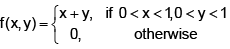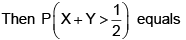Mathematical Statistics - 2017 Past Year Paper - Question 16

Let X1, X2, ..., Xm, Y1, Y2, ..., Yn be i.i.d. N(0, 1) random variables. Then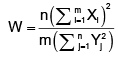has

Mathematical Statistics - 2017 Past Year Paper - Question 17be a sequence of i.i.d. random variables with the probability mass function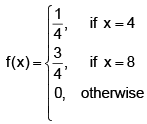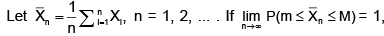then possible values of m and M are

Mathematical Statistics - 2017 Past Year Paper - Question 18

Let x1 = 1.1, x2 = 0.5, x3 = 1.4, x4 = 1.2 be the observed values of a random sample of size four from a distribution with the probability density function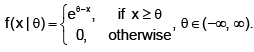Then the maximum likelihood estimate of θ2 is

Mathematical Statistics - 2017 Past Year Paper - Question 19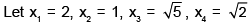be the observed values of a random sample of size four from a distribution with the probability density function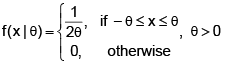Then the method of moments estimate of θ is

Mathematical Statistics - 2017 Past Year Paper - Question 20

Let X1, X2 be a random sample from an N(0, θ) distribution, where θ > 0. Then the value of k, for which the interval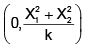is a 95% confidence interval for θ, equals

Mathematical Statistics - 2017 Past Year Paper - Question 21

Let X1, X2, X3, X4 be a random sample from N(θ1, σ2) distribution and Y1, Y2, Y3, Y4 be a random sample from N(θ1, σ2) distribution, where θ1, θ2 ∈ (-∞, ∞) and σ > 0. Further suppose that the two random samples are independent. For testing the null hypothesis H0 : θ1 = θ2 against the alternative hypothesis H1 : θ1 > θ2, suppose that a test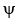rejects H0 if and only if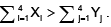The power of the tes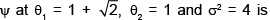Mathematical Statistics - 2017 Past Year Paper - Question 22

Let X be a random variable having a probability density function f ∈ {f0, f1}, where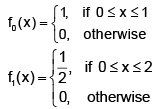For testing the null hypothesis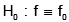against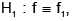based on a single observation on X, the power of the most powerful test of size α = 0.05 equals

Mathematical Statistics - 2017 Past Year Paper - Question 23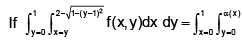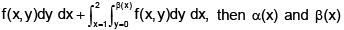are

Mathematical Statistics - 2017 Past Year Paper - Question 24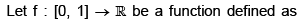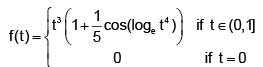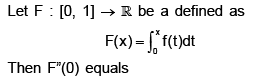Mathematical Statistics - 2017 Past Year Paper - Question 25

Consider the function

f(x, y) = x3 – y3 – 3x2 + 3y2 + 7, x,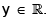Then the local minimum (m) and the local maximum (M) of f are given by

Mathematical Statistics - 2017 Past Year Paper - Question 26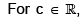let the sequence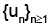be defined by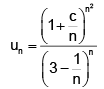Then the values of c for which the series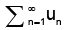converges are

Mathematical Statistics - 2017 Past Year Paper - Question 27

If for a suitable α > 0,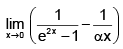exists and is equal to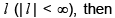Mathematical Statistics - 2017 Past Year Paper - Question 28

Let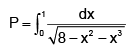Which of the following statements is TRUE ?

Mathematical Statistics - 2017 Past Year Paper - Question 29

Let Q, A, B be matrices of order n × n with real entries such that Q is orthogonal and A is invertible. Then the eigenvalues of QT A–1 BQ are always the same as those of

Mathematical Statistics - 2017 Past Year Paper - Question 30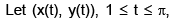be the curve defined by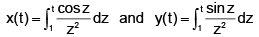Let L be the length of the arc of this curve from the origin to the point P on the curve at which the tangent is perpendicular to the x- axis. Then L equals

*Multiple options can be correct
Mathematical Statistics - 2017 Past Year Paper - Question 31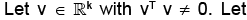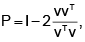where I is the k × k identity matrix. Then which of the following statements is (are) TRUE ?

*Multiple options can be correct
Mathematical Statistics - 2017 Past Year Paper - Question 32

Let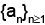and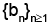be sequence of real numbers such thatis increasing andis decreasing. Under which of the following conditions, the sequence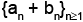is always convergent ?

*Multiple options can be correct
Mathematical Statistics - 2017 Past Year Paper - Question 33

Let f : [0, 1] → [0, 1] be defined as follows :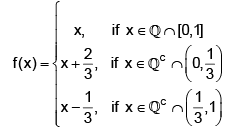Which of the following statements is (are) TRUE ?

*Multiple options can be correct
Mathematical Statistics - 2017 Past Year Paper - Question 34

Let f(x) be a non- negative differentiable function on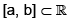such that f(a) = 0 = f(b) and |f’(x)| < 4. Let L1 and L2 be the straight lines given by the equations y = 4(x – a) and y = –4(x – b), respectively. Then which of the following statements is (are TRUe ?

*Multiple options can be correct
Mathematical Statistics - 2017 Past Year Paper - Question 35

Let E and F be two events with 0 < P(E) < 1, 0 < P(F) < 1 and P(E) + P(F) > 1. Which of the following statements is (are) TRUE ?

*Multiple options can be correct
Mathematical Statistics - 2017 Past Year Paper - Question 36

The cumulative distribution function of a random variable X is given by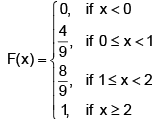Which of the following statements is (are) TRUE ?

*Multiple options can be correct
Mathematical Statistics - 2017 Past Year Paper - Question 37

Let X1, X2 be a random sample from a distribution with the probability mass function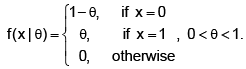Which of the following is (are) unbiased estimator(s) of θ?

*Multiple options can be correct
Mathematical Statistics - 2017 Past Year Paper - Question 38

Let X1, X2, X3 be a random sample from a distribution with the probability density function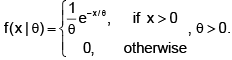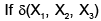is an unbiased estimator of θ, which of the following CANNOT be attained as a value of the variance of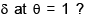*Multiple options can be correct
Mathematical Statistics - 2017 Past Year Paper - Question 39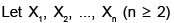be a random sample from a distribution with the probability density function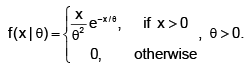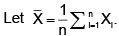Which of the following statistics is (are) sufficient but NOT complete ?

*Multiple options can be correct
Mathematical Statistics - 2017 Past Year Paper - Question 40

Let X1, X2, X3, X4 be a random sample from an N(θ, 1) distribution, where θ ∈ (-∞, ∞). Suppose the null hypothesis H0 : θ = 1 is to be tested against the hypothesis H1 : θ < 1 at α = 0.05 level of significance. For what observed values of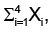the uniformly most powerful test would reject H0 ?

*Answer can only contain numeric values
Mathematical Statistics - 2017 Past Year Paper - Question 41

Let the random variable X have uniform distribution on the interval (0, 1) and Y = –2 loge X. Then E(Y) equals ________.

*Answer can only contain numeric values
Mathematical Statistics - 2017 Past Year Paper - Question 42

If Y = log10 X has N(μ, σ2) distribution with moment generating function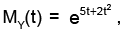, t ∈ (-∞, ∞), then P(X < 1000) equals ________.

*Answer can only contain numeric values
Mathematical Statistics - 2017 Past Year Paper - Question 43

Let X1, X2, X3, X4, X5 be independent random variables with X1 ~ N(200, 8), X2 ~ N(104, 8), X3 ~ N(108, 15), X4 ~ N(120, 15) and X5 ~ N(210, 15). Let U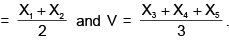Then P(U > V) equals _________.

*Answer can only contain numeric values
Mathematical Statistics - 2017 Past Year Paper - Question 44

Let X and Y be discrete random variables with the joint probability mass function.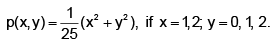Then P(Y = 1 | X = 1) equals _________.

*Answer can only contain numeric values
Mathematical Statistics - 2017 Past Year Paper - Question 45

Let X and Y be continuous random variables with the joint probability density function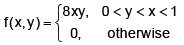Then 9Cov(X, Y) equals __________.

*Answer can only contain numeric values
Mathematical Statistics - 2017 Past Year Paper - Question 46

Let X1, X2, X3, Y1, Y2, Y3, Y4 be i.i.d. N(μ, σ2) random variables.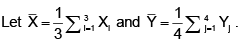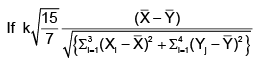has tv distribution, then (v – k) equals __________.

*Answer can only contain numeric values
Mathematical Statistics - 2017 Past Year Paper - Question 47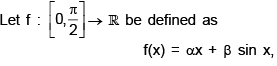where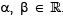. Let f have a local minimum at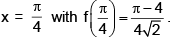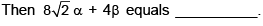*Answer can only contain numeric values
Mathematical Statistics - 2017 Past Year Paper - Question 48

The area bounded between two parabolas y = x2 + 4 and y = –x2 + 6 is __________.

*Answer can only contain numeric values
Mathematical Statistics - 2017 Past Year Paper - Question 49

For j = 1, 2, ..., 5, let Pj be the matrix of order 5 × 5 obtained by replacing the jth column of the identity matrix of order 5 × 5 with the column vector v = (5 4 3 2 1)T. Then the determinant of the matrix product P1 P2 P3 P4 P5 is __________

*Answer can only contain numeric values
Mathematical Statistics - 2017 Past Year Paper - Question 50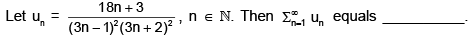*Answer can only contain numeric values
Mathematical Statistics - 2017 Past Year Paper - Question 51

Let a unit vector v = (v1 v2 v3)T be such that Av = 0 where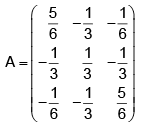Then the value of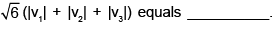*Answer can only contain numeric values
Mathematical Statistics - 2017 Past Year Paper - Question 52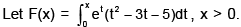Then the number of roots of F(x) = 0 in the interval (0, 4) is __________.

*Answer can only contain numeric values
Mathematical Statistics - 2017 Past Year Paper - Question 53

A tangent is drawn on the curve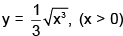at the point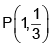which meets the x- axis
at Q. Then the length of the closed curve OQPO, where O is the origin, is __________.

*Answer can only contain numeric values
Mathematical Statistics - 2017 Past Year Paper - Question 54

The volume of the region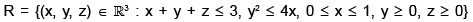is __________.

*Answer can only contain numeric values
Mathematical Statistics - 2017 Past Year Paper - Question 55

Let X be a continuous random variable with the probability density function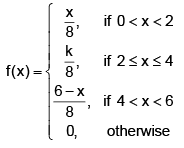where k is a real constant. Then P(1 < X < 5) equals __________.

*Answer can only contain numeric values
Mathematical Statistics - 2017 Past Year Paper - Question 56

Let X1, X2, X3 be independent random variables with the common probability density function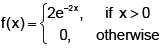Let Y = min {X1, X2, X3}, E(Y) =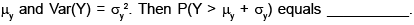*Answer can only contain numeric values
Mathematical Statistics - 2017 Past Year Paper - Question 57

Let X and Y be continuous random variables with the joint probability density function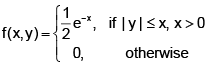Then E(X | Y = –1) equals __________.

*Answer can only contain numeric values
Mathematical Statistics - 2017 Past Year Paper - Question 58

Let X and Y be discrete random variables with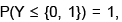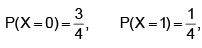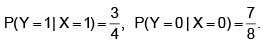Then 3P(Y = 1) – P(Y = 0) equals __________.

*Answer can only contain numeric values
Mathematical Statistics - 2017 Past Year Paper - Question 59

Let X1, X2, ..., X100 be i.i.d. random variables with E(X1) = 0, E(X12) = σ2, where s > 0. Let S =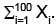If an approximate value of P(S < 30) is 0.9332, then σ2 equals __________.

*Answer can only contain numeric values
Mathematical Statistics - 2017 Past Year Paper - Question 60

Let X be a random variable with the probability density function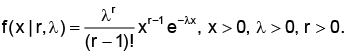If E(X) = 2 and Var(X) = 2, then P(X < 1) equals __________.

## IIT JAM Past Year Papers and Model Test Paper (All Branches)

8 docs|48 tests
Information about Mathematical Statistics - 2017 Past Year Paper Page
In this test you can find the Exam questions for Mathematical Statistics - 2017 Past Year Paper solved & explained in the simplest way possible. Besides giving Questions and answers for Mathematical Statistics - 2017 Past Year Paper, EduRev gives you an ample number of Online tests for practice

## IIT JAM Past Year Papers and Model Test Paper (All Branches)

8 docs|48 tests(Scan QR code)